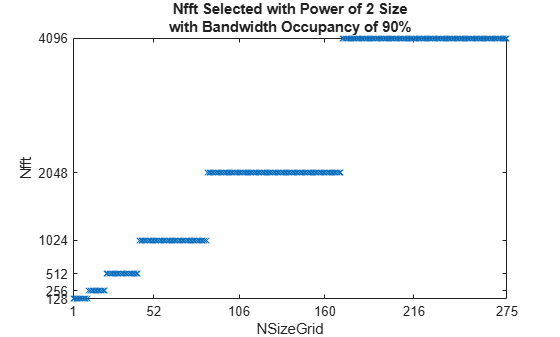# Use Custom OFDM Sample Rate and Custom FFT Size

This example shows how to set custom values for the sample rate input, `SampleRate`, and the fast Fourier transform (FFT) size input, `Nfft`, when you call an OFDM function (`nrOFDMModulate`, `nrOFDMInfo`, or `nrOFDMDemodulate`).

### Custom OFDM Sample Rate

The value that you set for the `SampleRate` input determines the sample rate of the waveform.

The nominal sample rate corresponding to the FFT size used in the OFDM modulation, ${\mathit{FFT}}_{\mathrm{SR}}$, is equal to `Nfft*carrier.SubcarrierSpacing*1000`, where `carrier` is the input argument of the function call that specifies the carrier configuration.

Because the resampling of the OFDM-modulated waveform is by a factor of `SampleRate/`${\mathit{FFT}}_{\mathrm{SR}}$, the resampling is costly if `SampleRate` and ${\mathit{FFT}}_{\mathrm{SR}}$ do not have large common factors.

### Custom FFT Size

The value that you set for the `Nfft` input must satisfy these conditions.

• `Nfft` is an integer (to ensure integer-valued cyclic prefix lengths).

• `Nfft` is a power of 2.

• `Nfft` results in a maximum occupancy of 100%. The actual occupancy is equal to `carrier.NSizeGrid*12/Nfft`.

You can only achieve a bandwidth occupancy of exactly 100% when these conditions apply.

• The FFT and the carrier grid have the same size. That is, `Nfft` is `carrier.NSizeGrid*12`.

• Resampling is not needed. That is, `SampleRate` is `Nfft*carrier.SubcarrierSpacing*1000`.

### Plot Bandwidth Occupancy

Create a carrier configuration object.

`carrier = nrCarrierConfig;`

Set the `SampleRate` for each `NSizeGrid` such that the bandwidth occupancy (`txBW/SampleRate`) is 90%. Set `Nfft` to a power of 2.

```nSizeGrids = 1:275; userSampleRateUserNfft = zeros(1,275); fftOccupancy = zeros(1,275); for nSizeGrid = 1:275 carrier.NSizeGrid = nSizeGrid; % Transmission bandwidth of OFDM waveform txBW = carrier.NSizeGrid*12*carrier.SubcarrierSpacing*1000; sr = txBW / 0.9; nfft = max(128,2^ceil(log2(carrier.NSizeGrid*12))); ofdmInfo = nrOFDMInfo(carrier,'SampleRate',sr,'Nfft',nfft); userSampleRateUserNfft(nSizeGrid) = ofdmInfo.Nfft; fftOccupancy(nSizeGrid) = carrier.NSizeGrid*12/ofdmInfo.Nfft; end```

Plot the resulting FFT size.

```figure; plot(nSizeGrids,userSampleRateUserNfft,'x'); title({'Nfft Selected with Power of 2 Size' 'with Bandwidth Occupancy of 90%'}); axis([1 275 min(userSampleRateUserNfft) max(userSampleRateUserNfft)]); xlabel('NSizeGrid'); xticks([1 52 106 160 216 275]); ylabel('Nfft'); yticks(2.^(7:12));```Plot the resulting FFT occupancy.

```figure; plot(nSizeGrids,fftOccupancy,'x'); title({'FFT Occupancy' 'with Bandwidth Occupancy of 90%'}); axis([1 275 0 1]); xlabel('NSizeGrid'); xticks([1 52 106 160 216 275]); ylabel('FFT Occupancy');```### Get OFDM Information

Update the carrier to 25 resource blocks (RBs).

`carrier.NSizeGrid = 25;`

Get OFDM information.

`ofdmInfo = nrOFDMInfo(carrier)`
```ofdmInfo = struct with fields: Nfft: 512 SampleRate: 7680000 CyclicPrefixLengths: [40 36 36 36 36 36 36 40 36 36 36 36 36 36] SymbolLengths: [552 548 548 548 548 548 548 552 548 548 548 548 548 548] Windowing: 18 SymbolPhases: [0 0 0 0 0 0 0 0 0 0 0 0 0 0] SymbolsPerSlot: 14 SlotsPerSubframe: 1 SlotsPerFrame: 10 ```

The `CyclicPrefixLengths` and `SymbolLengths` fields in the output structure return the cyclic prefix lengths and total OFDM symbol lengths for each OFDM symbol in a subframe. The total OFDM symbol length is composed of the cyclic prefix and the nominal OFDM symbol length equal to the FFT size.

`ofdmInfo.SymbolLengths - ofdmInfo.CyclicPrefixLengths`
```ans = 1×14 512 512 512 512 512 512 512 512 512 512 512 512 512 512 ```

Get OFDM information for a specified FFT size. Because OFDM symbol construction is performed using an IFFT of size that you specify in the `Nfft` input, the `CyclicPrefixLengths` and `SymbolLengths` fields return values in terms of this `Nfft` value. The specified `Nfft` value is also returned in the `Nfft` field of the output.

```nfft = 640; ofdmInfo = nrOFDMInfo(carrier,'Nfft',nfft)```
```ofdmInfo = struct with fields: Nfft: 640 SampleRate: 9600000 CyclicPrefixLengths: [50 45 45 45 45 45 45 50 45 45 45 45 45 45] SymbolLengths: [690 685 685 685 685 685 685 690 685 685 685 685 685 685] Windowing: 22 SymbolPhases: [0 0 0 0 0 0 0 0 0 0 0 0 0 0] SymbolsPerSlot: 14 SlotsPerSubframe: 1 SlotsPerFrame: 10 ```
`ofdmInfo.SymbolLengths - ofdmInfo.CyclicPrefixLengths`
```ans = 1×14 640 640 640 640 640 640 640 640 640 640 640 640 640 640 ```

Get OFDM information for a specified FFT size and sample rate. For the default sample rate, the number of time-domain samples of each OFDM symbol matches the values of the `SymbolLengths` field. If you specify the `SampleRate` input, the specified value is returned in the `SampleRate` field of the output. However, because of the `CyclicPrefixLengths` and `SymbolLengths` fields are expressed in terms of the IFFT size used during OFDM symbol construction, these values do not change in the output. The waveform is resampled to the sample rate that you specify after OFDM symbol construction. Depending on the sample rate, the length of the cyclic prefixes and nominal OFDM symbols in the corresponding OFDM waveform might not be an integer number of samples.

```sr = 1.35 * nfft * carrier.SubcarrierSpacing * 1e3; ofdmInfo = nrOFDMInfo(carrier,'Nfft',640,'SampleRate',sr)```
```ofdmInfo = struct with fields: Nfft: 640 SampleRate: 12960000 CyclicPrefixLengths: [50 45 45 45 45 45 45 50 45 45 45 45 45 45] SymbolLengths: [690 685 685 685 685 685 685 690 685 685 685 685 685 685] Windowing: 22 SymbolPhases: [0 0 0 0 0 0 0 0 0 0 0 0 0 0] SymbolsPerSlot: 14 SlotsPerSubframe: 1 SlotsPerFrame: 10 ```
`ratio = ofdmInfo.SampleRate / (ofdmInfo.Nfft * carrier.SubcarrierSpacing * 1e3)`
```ratio = 1.3500 ```
`disp(num2str(ofdmInfo.SymbolLengths*ratio,'%0.3f '));`
```931.500 924.750 924.750 924.750 924.750 924.750 924.750 931.500 924.750 924.750 924.750 924.750 924.750 924.750 ```